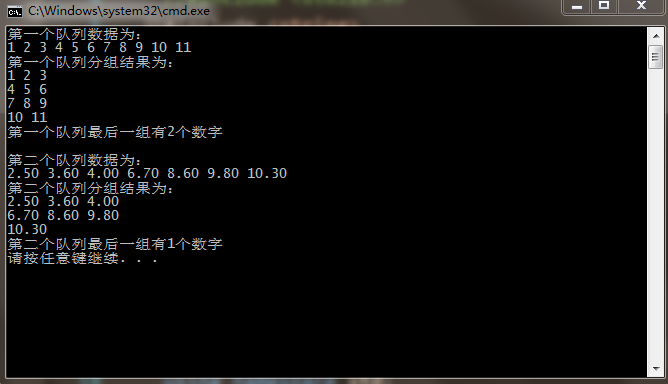C编程问题求解，求指点，

4个回答

``````#include <stdio.h>
int main()
{
int queueArray1[] = { 1,2,3,4,5,6,7,8,9,10,11};
double  queueArray2[] = {2.5,3.6,4.0,6.7,8.6,9.8,10.3};

int lenArray1 = sizeof(queueArray1) / sizeof(int);
int lenArray2 = sizeof(queueArray2) / sizeof(double);

printf("第一个队列数据为：\n");
for (int i = 0; i < lenArray1; i++)
{
printf("%d ", queueArray1[i]);
}

printf("\n第一个队列分组结果为：\n");

for (int i = 0; i < lenArray1; i++)
{
if ( i%3 ==0 && i >2 )
{
printf("\n");
}
printf("%d ", queueArray1[i]);

}
printf("\n第一个队列最后一组有%d个数字\n\n", lenArray1%3 == 0 ? 3:  lenArray1 % 3);

printf("第二个队列数据为：\n");
for (int i = 0; i < lenArray2; i++)
{
printf("%3.2f ", queueArray2[i]);
}

printf("\n第二个队列分组结果为：\n");

for (int i = 0; i < lenArray2; i++)
{
if (i % 3 == 0 && i >2)
{
printf("\n");
}
printf("%3.2f ", queueArray2[i]);

}
printf("\n第二个队列最后一组有%d个数字\n", lenArray2 % 3 == 0 ? 3 :  lenArray2 % 3);

return 0;
}
``````itoa转换成字符串，求长度，判断是否能被3整除